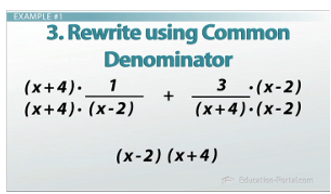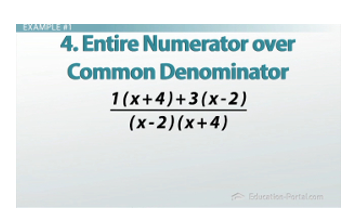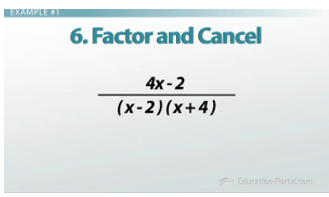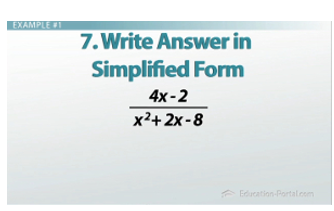# How to Add and Subtract Rational Expressions

#### Video Lesson on How to Add and Subtract Rational Expressions

This lesson video Not available at this time available video coming soon

### How to Add and Subtract Rational Expressions

Adding and subtracting rational expressions brings everything you learned about fractions into the world of algebra. We will mix common denominators with factoring and FOILing.

### Rational Polynomial Defined

The word 'rational' means 'fraction.' So a rational polynomial is a fraction with polynomials in the numerator (top) and/or denominator (bottom). Here's an example of a rational polynomial:

(x + 4) / (x^2 + 3x + 2)

As we get started, let's remember that to add or subtract fractions, we need a common denominator. Try this mnemonic to help you remember when you need a common denominator and when you don't:

Add Subtract Common Denominators; Multiply Divide None

Auntie sits counting diamonds; Mother does not.

Let's get started!

### Adding and Subtracting Rational Expressions

1. We need to factor.
2. Find a common denominator.
3. Rewrite each fraction using the common denominator.
4. Put the entire numerator over the common denominator.
5. Simplify the numerator.
6. Factor and cancel if possible.
7. Write the final answer in simplified form.

There are quite a few steps, but let me show you how they workFind the common denominator and use it to rewrite the fractions

### Example #1

Our first expression is (1 / (x - 2)) + (3 / (x + 4)).

The first step is to factor. Since we don't have anything to factor, let's move to the next step, writing down our denominators, (x-2) and (x+4). This will be our common denominator: (x - 2)(x + 4).

Now we need to create our common denominator. Let's look at our first term, (1 / (x - 2)). (x - 2) is in the denominator. We need to multiply by (x + 4) to make our common denominator. But if we multiply by (x + 4) on the bottom, we need to multiply by (x + 4) on the top.

For right now we are going to write it and not multiply yet.

Let's look at our second term: (3 / (x + 4)). The denominator is (x + 4). We need to multiply (x - 2) times (x + 4) to get our common denominator. But once again, if we multiply by (x - 2) on the bottom, we need to multiply by it on the top too.

So far, this is what we have:

((1(x + 4)) / ((x - 2)(x + 4))) + ((3(x - 2)) / ((x + 4)(x - 2)))

Don't FOIL the denominator - we may have to cancel as our final answer!

Now let's write the entire numerator over our common denominator.

(1(x + 4)) + 3(x - 2)) / ((x - 2)(x + 4))

Let's simplify the numerator.

1(x + 4) = x + 4

3(x - 2) = 3x – 6

(x + 4 + 3x - 6) / ((x + 4)(x - 2))

Collect like terms in the numerator.Putting the numerator over the common denominator prepares the problem for simplifying

(4x - 2) / ((x + 4)(x - 2))

Factor the numerator if possible.

4x - 2 = 2 (2x - 1)

(2(2x - 1)) / ((x + 4)(x - 2))

There isn't anything to slash or cancel, so we distribute and FOIL for our final answer.

(4x - 2) / (x^2 + 2x - 8)

### Example #2

((2x) / (x^2 - 16)) - (1 / (x + 4))

x^2 - 16 factors into (x - 4)(x + 4). So let's put that into the expression.

((2x) / ((x - 4)(x + 4))) - (1 /(x + 4))

Our next step is to write down all of our denominators.

In the first term, we have (x + 4)(x - 4), so we write those down.

We continue to the next term and look at the denominator. We never duplicate denominators from term to term. Since we already have (x + 4) written as part of our denominator, we don't need to duplicate it. So it turns out our common denominator will be (x + 4)(x - 4).

Now we need to create our common denominator. Let's look at our first term ((2x) / (x + 4)(x - 4)). We already have our common denominator here, so we're going to move to the next term: (1 / (x + 4)).

Here, we need to multiply (x - 4) to make our common denominator. But if we multiply (x - 4) on the bottom, we need to multiply by (x - 4) on the top. For right now, we are going to write it and not multiply yet. So we have ((2x) / (x + 4)(x - 4)) - (1(x - 4) / (x + 4)(x - 4)).After collecting like terms, the resulting expression can be factored

Let's write the numerator all over the denominator.

((2x)-1(x-4))/((x+ 4)(x - 4))

Simplify the numerator (or top) and rewrite it over the denominator.

Distribute the -1 into (x - 4) = -1x + 4.

Collecting like terms, 2x - 1x= x.

So now our expression looks like:

(x + 4) / (x+ 4)(x- 4)

We can slash, or cancel, (x+ 4) over (x+ 4).

This gives us 1/(x - 4) as our final answer.

### Example #3

((5x^2 - 3) / (x^2 + 6x + 8)) – 4

The first step is to factor.

x^2 + 6x + 8 = (x + 4)(x + 2)

Our next step is to write down all of our denominators.

In our first term, we have (x + 4)(x + 2), so we write it down.

The denominator for the next term is 1.

Therefore, our common denominator will be (x + 4)(x + 2).

Now we need to create our common denominator. Let's look at our first term (5x^2 - 3)/((x + 4)(x + 2)).

We already have our common denominator here, so we're going to move to the next term, 4.

Here, we only have a 1 in the denominator, so we need to multiply by (x + 4)(x + 2) over (x+4)(x+2).

This is what our new expression is going to look like:

((5x^2 - 3) / (x + 4)(x + 2)) - ((4 (x + 4)(x + 2)) / ((x + 4)(x + 2))) .The final step after using FOIL to simplify the expression

Let's write the whole numerator (top) over the denominator (bottom).

((5x^2 - 3 - 4(x + 4)(x + 2))) / ((x + 4)(x + 2))

We can now simplify the top, or numerator.

(x+4)(x+2) = x^2 +6x +8

Multiply -4( x^2 +6x +8) and we have -4x^2 - 24x - 32.

Let's continue with the numerator and collect like terms, so our expression looks like:

(x^2 - 24x - 35) / ((x + 4)(x + 2))

The numerator does not factor without using the quadratic formula, so this is almost our answer, except we need to FOIL the bottom, or denominator. Here is our final answer:

(x^2 - 24x - 35) / (x^2 + 6x + 8)

### Lesson Summary

As we have seen, the process to add or subtract rational expressions is:

1. We need to factor.
2. Find a common denominator.
3. Rewrite each fraction using the common denominator.
4. Put the entire numerator over the common denominator.
5. Simplify the numerator.
6. Factor and cancel if possible.
7. Write the final answer in simplified form.

### Next Topics